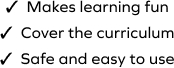# Solve Word Problems on Decimal Multiplication

SplashLearn - The complete PreK - Grade 5 Math & ELA Learning Program Built for Your Child
Home > Solve Word Problems on Decimal Multiplication
The game gives students an opportunity to apply the concepts of decimal multiplication to the real world. Students will work with a set of decimal word problems. They will make sense of "multiply by" scenarios and find the final answer. Students will choose the correct answer from the given options to solve the problems.